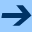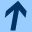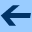On covariant embeddings of a linear functional equation with respect to an analytic iteration group in some non-generic cases.

## On covariant embeddings of a linear functional equation with respect to an analytic iteration group in some non-generic cases.

Jointly written with LUDWIG REICH.

Aequationes mathematicae 68, 60 - 87, 2004.

Abstract: In the paper On covariant embeddings of a linear functional equation with respect to an analytic iteration group the authors described the problem of covariant embeddings of a linear functional equation with respect to analytic iteration groups and solved it in the generic cases. However, some cases remained unsolved. In this paper we present the solution for these open cases.

harald.fripertinger "at" uni-graz.at, March 4, 2019